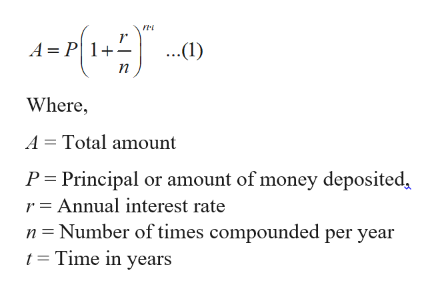# Determine the amount of money you will have 10 years if you deposit \$8,000 at 5.6% annual interest compound quarterly, using A= P(1 +r/n)nt round to the nearest cent.

Question
6 views

Determine the amount of money you will have 10 years if you deposit \$8,000 at 5.6% annual interest compound quarterly, using A= P(1 +r/n)nt round to the nearest cent.

check_circle

Step 1

To find amount we u...help_outlineImage Transcriptionclose-r A P 1 ..(1) Where, A Total amount P Principal or amount of money deposited r = Annual interest rate n Number of times compounded per year t = Time in years fullscreen

### Want to see the full answer?

See Solution

#### Want to see this answer and more?

Solutions are written by subject experts who are available 24/7. Questions are typically answered within 1 hour.*

See Solution
*Response times may vary by subject and question.
Tagged in

### Algebra